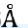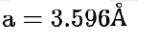# The unit cell of copper corresponds to a face centered cube of edge lengthQuestion:

The unit cell of copper corresponds to a face centered cube of edge length 3.596with one copper atom at each lattice point. The calculated density of copper in $\mathrm{kg} / \mathrm{m}^{3}$ is_______________ . [Molar mass of Cu: $63.54 \mathrm{~g} ;$ Avogadro number $\left.=6.022 \times 10^{23}\right]$

Solution:

(9077)$\mathrm{d}=\frac{Z \times G M M}{N_{A} \times a^{3}}$

$\mathrm{d}=\frac{4 \times 63.54 \times 10^{-3}}{6.022 \times 10^{23} \times\left(3.596 \times 10^{-10}\right)^{3}}$

$\mathrm{d}=0.9076 \times 10^{4}=9076.2 \mathrm{~kg} / \mathrm{m}^{3}$